# Midpoint theorem examples

Example 1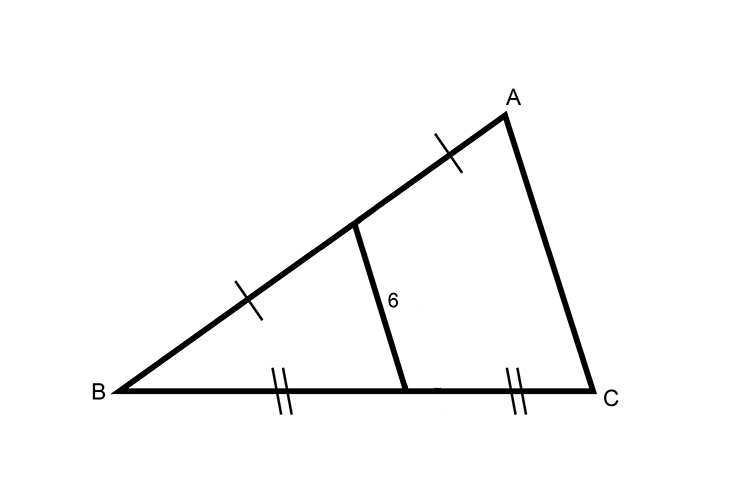Find the distance AC

The lines BC and BA are bisected at their midpointsXY must therefore be parallel to AC

And midpoint theorem tells us that

XY is half the length of AC

Therefore

AC=2times6

AC=12

Example 2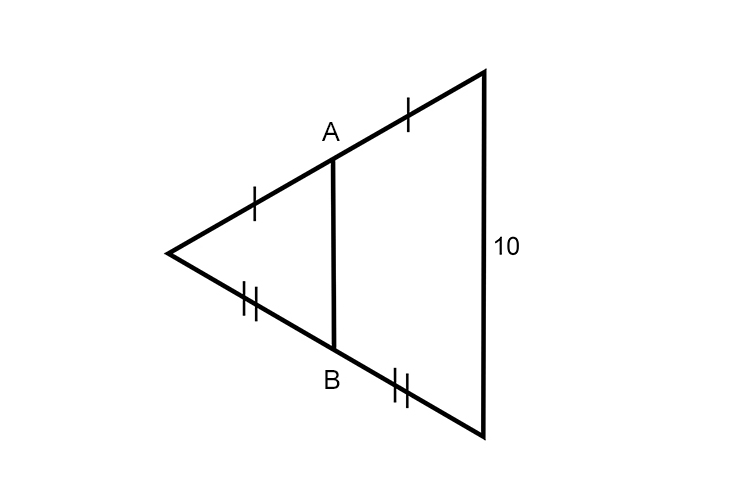Find the distance AB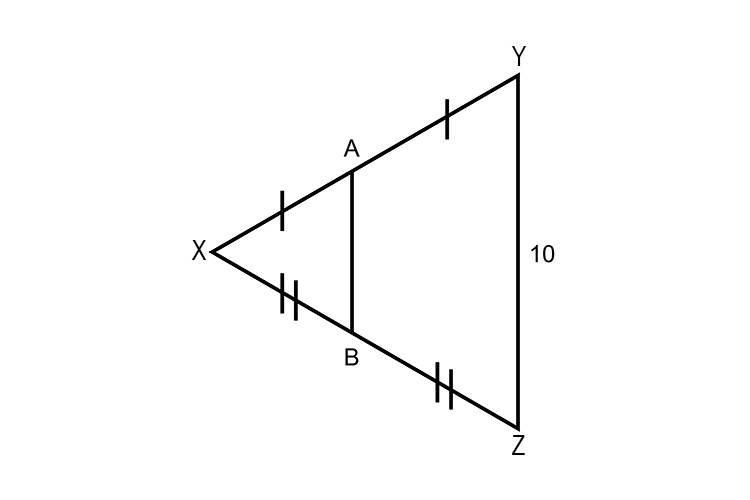The lines XY and XZ are bisected at their midpoints

Therefore AB and YZ must be parallel

And midpoint theorem tells us that

AB is half the length of YZ

Therefore

AB=5

Example 3

Find the distance XY in the following diagram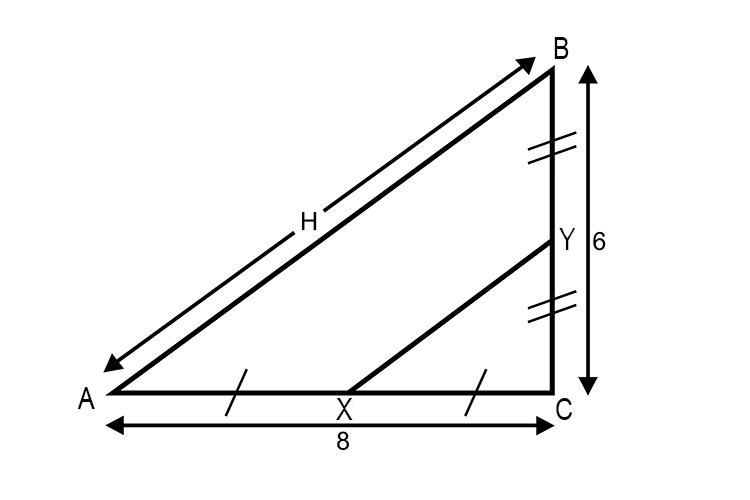Solution

Using Pythagoras theorem 8^2+6^2=H^2

Therefore

H^2=64+36

H^2=100

H=10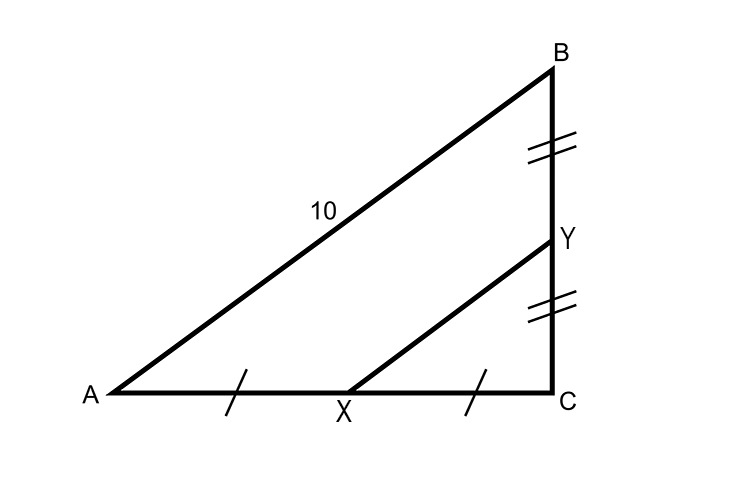The lines AC and CB are bisected at their midpoints

XY must therefore be parallel to AB

And midpoints theorem tells us that

XY is half the length of AB

Therefore XY is 1/2times 10

Answer: XY=5